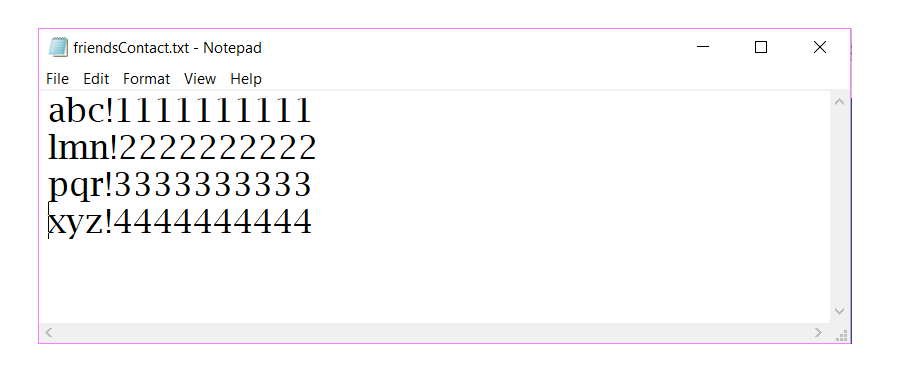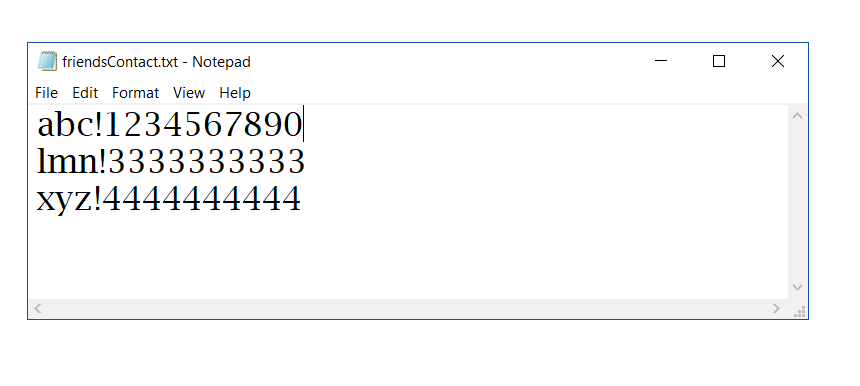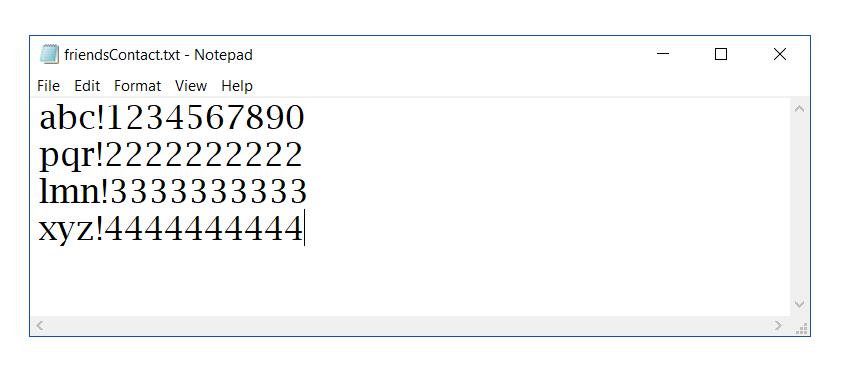Related Articles

# File Handling in Java with CRUD operations

• Difficulty Level : Hard
• Last Updated : 08 Mar, 2021

So far the operations using Java programs are done on a prompt/terminal which is not stored anywhere. But in the software industry, most of the programs are written to store the information fetched from the program. One such way is to store the fetched information in a file.

What is File Handling in Java?

A file is a container that is used to store various types of information. Data is permanently stored in secondary memory by creating a file with a unique name. A file may consist of text, image or any other document.

Different operations that can be performed on a file are:

1. Creation of a new file
2. Opening an existing file
4. Writing to a file
5. Moving to a specific location in a file
6. Closing a file

Different classes that can be used in Java for File Handling:

In this article, we will learn how Java ByteStreams are used to perform input and output of 8-bits(1 byte) using class RandomAccessFile which consists of method writeBytes() and readBytes() to write and read the data in the form of bytes.

Various Methods used to perform File operation:

• writeBytes(String s): Writes the string to the file as a sequence of bytes.
• getFilePointer(): Returns the current offset in this file.
• length(): Returns the length of this file and return type is long.
• close(): Closes this random access file stream and releases any system resources associated with the stream.
• setLength(long newLength): Sets the length of this file.
• seek(long pos): Sets the file-pointer offset, measured from the beginning of this file, at which the next read or write occurs.

File opening modes in Java:

Syntax of Opening File using RandomAccessFile:

```File file = new File( filename )
RandomAccessFile raf = new RandomAccessFile(file, mode)```

CRUD operations using File Handling in Java

Example: Consider that you want to keep records of your friend’s contact number in a file. To distinguish between your friend’s name and contact number you need a separator. To do, so you need to select a separator such as ‘!’ or ‘\$’ or some special symbol which does not appear in your friend’s name. Then we will form a string consist of a name, special symbol and number to insert into the file.

Syntax of a Contact in the file friendsContact.txt:

`Name!Number`

How to Create a File in Java?

## Java

 `// Java program to create a file "friendsContact.txt"``// and add a new contact in the file` `import` `java.io.File;``import` `java.io.IOException;``import` `java.io.RandomAccessFile;``import` `java.lang.NumberFormatException;` `class` `AddFriend {` `    ``public` `static` `void` `main(String data[])``    ``{` `        ``try` `{` `            ``// Get the name of the contact to be updated``            ``// from the Command line argument``            ``String newName = data[``0``];` `            ``// Get the number to be updated``            ``// from the Command line argument``            ``long` `newNumber = Long.parseLong(data[``1``]);` `            ``String nameNumberString;``            ``String name;``            ``long` `number;``            ``int` `index;` `            ``// Using file pointer creating the file.``            ``File file = ``new` `File(``"friendsContact.txt"``);` `            ``if` `(!file.exists()) {` `                ``// Create a new file if not exists.``                ``file.createNewFile();``            ``}` `            ``// Opening file in reading and write mode.` `            ``RandomAccessFile raf``                ``= ``new` `RandomAccessFile(file, ``"rw"``);``            ``boolean` `found = ``false``;` `            ``// Checking whether the name``            ``// of contact already exists.``            ``// getFilePointer() give the current offset``            ``// value from start of the file.``            ``while` `(raf.getFilePointer() < raf.length()) {` `                ``// reading line from the file.``                ``nameNumberString = raf.readLine();` `                ``// splitting the string to get name and``                ``// number``                ``String[] lineSplit``                    ``= nameNumberString.split(``"!"``);` `                ``// separating name and number.``                ``name = lineSplit[``0``];``                ``number = Long.parseLong(lineSplit[``1``]);` `                ``// if condition to find existence of record.``                ``if` `(name == newName``                    ``|| number == newNumber) {``                    ``found = ``true``;``                    ``break``;``                ``}``            ``}` `            ``if` `(found == ``false``) {` `                ``// Enter the if block when a record``                ``// is not already present in the file.``                ``nameNumberString``                    ``= newName + ``"!"``                      ``+ String.valueOf(newNumber);` `                ``// writeBytes function to write a string``                ``// as a sequence of bytes.``                ``raf.writeBytes(nameNumberString);` `                ``// To insert the next record in new line.``                ``raf.writeBytes(System.lineSeparator());` `                ``// Print the message``                ``System.out.println(``" Friend added. "``);` `                ``// Closing the resources.``                ``raf.close();``            ``}``            ``// The contact to be updated``            ``// could not be found``            ``else` `{` `                ``// Closing the resources.``                ``raf.close();` `                ``// Print the message``                ``System.out.println(``" Input name"``                                   ``+ ``" does not exists. "``);``            ``}``        ``}` `        ``catch` `(IOException ioe) {` `            ``System.out.println(ioe);``        ``}``        ``catch` `(NumberFormatException nef) {` `            ``System.out.println(nef);``        ``}``    ``}``}`

Output:

Compiling and Adding the contact in the newly created file:

```javac AddFriend.java

Input name or number already exist```

File:How to Read a File in Java?

## Java

 `// Java program to read from file "friendsContact.txt"``// and display the contacts` `import` `java.io.File;``import` `java.io.IOException;``import` `java.io.RandomAccessFile;``import` `java.lang.NumberFormatException;` `class` `DisplayFriends {` `    ``public` `static` `void` `main(String data[])``    ``{` `        ``try` `{` `            ``String nameNumberString;``            ``String name;``            ``long` `number;``            ``int` `index;` `            ``// Using file pointer creating the file.``            ``File file = ``new` `File(``"friendsContact.txt"``);` `            ``if` `(!file.exists()) {` `                ``// Create a new file if not exists.``                ``file.createNewFile();``            ``}` `            ``// Opening file in reading and write mode.` `            ``RandomAccessFile raf``                ``= ``new` `RandomAccessFile(file, ``"rw"``);``            ``boolean` `found = ``false``;` `            ``// Traversing the file``            ``// getFilePointer() give the current offset``            ``// value from start of the file.``            ``while` `(raf.getFilePointer() < raf.length()) {` `                ``// reading line from the file.``                ``nameNumberString = raf.readLine();` `                ``// splitting the string to get name and``                ``// number``                ``String[] lineSplit``                    ``= nameNumberString.split(``"!"``);` `                ``// separating name and number.``                ``name = lineSplit[``0``];``                ``number = Long.parseLong(lineSplit[``1``]);` `                ``// Print the contact data``                ``System.out.println(``                    ``"Friend Name: "` `+ name + ``"\n"``                    ``+ ``"Contact Number: "` `+ number + ``"\n"``);``            ``}` `            ``catch` `(IOException ioe)``            ``{` `                ``System.out.println(ioe);``            ``}``            ``catch` `(NumberFormatException nef)``            ``{` `                ``System.out.println(nef);``            ``}``        ``}``    ``}`

Output:

Compiling and reading the contacts from the file:

```javac DisplayFriends.java

java DisplayFriends
Friend Name: abc
Contact Number: 1234567890

Friend Name: lmn
Contact Number: 3333333333

Friend Name: xyz
Contact Number: 4444444444```

File:How to Update a File in Java?

## Java

 `// Java program to update in the file "friendsContact.txt"``// and change the number of an old contact` `import` `java.io.File;``import` `java.io.IOException;``import` `java.io.RandomAccessFile;``import` `java.lang.NumberFormatException;` `class` `UpdateFriend {` `    ``public` `static` `void` `main(String data[])``    ``{` `        ``try` `{` `            ``// Get the name of the contact to be updated``            ``// from the Command line argument``            ``String newName = data[``0``];` `            ``// Get the number to be updated``            ``// from the Command line argument``            ``long` `newNumber = Long.parseLong(data[``1``]);` `            ``String nameNumberString;``            ``String name;``            ``long` `number;``            ``int` `index;` `            ``// Using file pointer creating the file.``            ``File file = ``new` `File(``"friendsContact.txt"``);` `            ``if` `(!file.exists()) {` `                ``// Create a new file if not exists.``                ``file.createNewFile();``            ``}` `            ``// Opening file in reading and write mode.``            ``RandomAccessFile raf``                ``= ``new` `RandomAccessFile(file, ``"rw"``);``            ``boolean` `found = ``false``;` `            ``// Checking whether the name``            ``// of contact already exists.``            ``// getFilePointer() give the current offset``            ``// value from start of the file.``            ``while` `(raf.getFilePointer() < raf.length()) {` `                ``// reading line from the file.``                ``nameNumberString = raf.readLine();` `                ``// splitting the string to get name and``                ``// number``                ``String[] lineSplit``                    ``= nameNumberString.split(``"!"``);` `                ``// separating name and number.``                ``name = lineSplit[``0``];``                ``number = Long.parseLong(lineSplit[``1``]);` `                ``// if condition to find existence of record.``                ``if` `(name == newName``                    ``|| number == newNumber) {``                    ``found = ``true``;``                    ``break``;``                ``}``            ``}` `            ``// Update the contact if record exists.``            ``if` `(found == ``true``) {` `                ``// Creating a temporary file``                ``// with file pointer as tmpFile.``                ``File tmpFile = ``new` `File(``"temp.txt"``);` `                ``// Opening this temporary file``                ``// in ReadWrite Mode``                ``RandomAccessFile tmpraf``                    ``= ``new` `RandomAccessFile(tmpFile, ``"rw"``);` `                ``// Set file pointer to start``                ``raf.seek(``0``);` `                ``// Traversing the friendsContact.txt file``                ``while` `(raf.getFilePointer()``                       ``< raf.length()) {` `                    ``// Reading the contact from the file``                    ``nameNumberString = raf.readLine();` `                    ``index = nameNumberString.indexOf(``'!'``);``                    ``name = nameNumberString.substring(``                        ``0``, index);` `                    ``// Check if the fetched contact``                    ``// is the one to be updated``                    ``if` `(name.equals(inputName)) {` `                        ``// Update the number of this contact``                        ``nameNumberString``                            ``= name + ``"!"``                              ``+ String.valueOf(newNumber);``                    ``}` `                    ``// Add this contact in the temporary``                    ``// file``                    ``tmpraf.writeBytes(nameNumberString);` `                    ``// Add the line separator in the``                    ``// temporary file``                    ``tmpraf.writeBytes(``                        ``System.lineSeparator());``                ``}` `                ``// The contact has been updated now``                ``// So copy the updated content from``                ``// the temporary file to original file.` `                ``// Set both files pointers to start``                ``raf.seek(``0``);``                ``tmpraf.seek(``0``);` `                ``// Copy the contents from``                ``// the temporary file to original file.``                ``while` `(tmpraf.getFilePointer()``                       ``< tmpraf.length()) {``                    ``raf.writeBytes(tmpraf.readLine());``                    ``raf.writeBytes(System.lineSeparator());``                ``}` `                ``// Set the length of the original file``                ``// to that of temporary.``                ``raf.setLength(tmpraf.length());` `                ``// Closing the resources.``                ``tmpraf.close();``                ``raf.close();` `                ``// Deleting the temporary file``                ``tmpFile.delete();` `                ``System.out.println(``" Friend updated. "``);``            ``}` `            ``// The contact to be updated``            ``// could not be found``            ``else` `{` `                ``// Closing the resources.``                ``raf.close();` `                ``// Print the message``                ``System.out.println(``" Input name"``                                   ``+ ``" does not exists. "``);``            ``}``        ``}` `        ``catch` `(IOException ioe) {``            ``System.out.println(ioe);``        ``}` `        ``catch` `(NumberFormatException nef) {``            ``System.out.println(nef);``        ``}``    ``}``}`

Output:

Compiling and updating the contact in the file:

```javac UpdateFriend.java

java UpdateFriend abc 1234567890
Friend updated.

java UpdateFriend tqr
Input name does not exists.```

File:How to Delete a File in Java?

## Java

 `// Java program to delete a contact``// from the file "friendsContact.txt"` `import` `java.io.File;``import` `java.io.IOException;``import` `java.io.RandomAccessFile;``import` `java.lang.NumberFormatException;` `class` `DeleteFriend {` `    ``public` `static` `void` `main(String data[])``    ``{` `        ``try` `{` `            ``// Get the name of the contact to be updated``            ``// from the Command line argument``            ``String newName = data[``0``];` `            ``String nameNumberString;``            ``String name;``            ``long` `number;``            ``int` `index;` `            ``// Using file pointer creating the file.``            ``File file = ``new` `File(``"friendsContact.txt"``);` `            ``if` `(!file.exists()) {` `                ``// Create a new file if not exists.``                ``file.createNewFile();``            ``}` `            ``// Opening file in reading and write mode.``            ``RandomAccessFile raf``                ``= ``new` `RandomAccessFile(file, ``"rw"``);``            ``boolean` `found = ``false``;` `            ``// Checking whether the name of contact exists.``            ``// getFilePointer() give the current offset``            ``// value from start of the file.``            ``while` `(raf.getFilePointer() < raf.length()) {` `                ``// reading line from the file.``                ``nameNumberString = raf.readLine();` `                ``// splitting the string to get name and``                ``// number``                ``String[] lineSplit``                    ``= nameNumberString.split(``"!"``);` `                ``// separating name and number.``                ``name = lineSplit[``0``];``                ``number = Long.parseLong(lineSplit[``1``]);` `                ``// if condition to find existence of record.``                ``if` `(name == newName) {``                    ``found = ``true``;``                    ``break``;``                ``}``            ``}` `            ``// Delete the contact if record exists.``            ``if` `(found == ``true``) {` `                ``// Creating a temporary file``                ``// with file pointer as tmpFile.``                ``File tmpFile = ``new` `File(``"temp.txt"``);` `                ``// Opening this temporary file``                ``// in ReadWrite Mode``                ``RandomAccessFile tmpraf``                    ``= ``new` `RandomAccessFile(tmpFile, ``"rw"``);` `                ``// Set file pointer to start``                ``raf.seek(``0``);` `                ``// Traversing the friendsContact.txt file``                ``while` `(raf.getFilePointer()``                       ``< raf.length()) {` `                    ``// Reading the contact from the file``                    ``nameNumberString = raf.readLine();` `                    ``index = nameNumberString.indexOf(``'!'``);``                    ``name = nameNumberString.substring(``                        ``0``, index);` `                    ``// Check if the fetched contact``                    ``// is the one to be deleted``                    ``if` `(name.equals(inputName)) {` `                        ``// Skip inserting this contact``                        ``// into the temporary file``                        ``continue``;``                    ``}` `                    ``// Add this contact in the temporary``                    ``// file``                    ``tmpraf.writeBytes(nameNumberString);` `                    ``// Add the line separator in the``                    ``// temporary file``                    ``tmpraf.writeBytes(``                        ``System.lineSeparator());``                ``}` `                ``// The contact has been deleted now``                ``// So copy the updated content from``                ``// the temporary file to original file.` `                ``// Set both files pointers to start``                ``raf.seek(``0``);``                ``tmpraf.seek(``0``);` `                ``// Copy the contents from``                ``// the temporary file to original file.``                ``while` `(tmpraf.getFilePointer()``                       ``< tmpraf.length()) {``                    ``raf.writeBytes(tmpraf.readLine());``                    ``raf.writeBytes(System.lineSeparator());``                ``}` `                ``// Set the length of the original file``                ``// to that of temporary.``                ``raf.setLength(tmpraf.length());` `                ``// Closing the resources.``                ``tmpraf.close();``                ``raf.close();` `                ``// Deleting the temporary file``                ``tmpFile.delete();` `                ``System.out.println(``" Friend deleted. "``);``            ``}` `            ``// The contact to be deleted``            ``// could not be found``            ``else` `{` `                ``// Closing the resources.``                ``raf.close();` `                ``// Print the message``                ``System.out.println(``" Input name"``                                   ``+ ``" does not exists. "``);``            ``}``        ``}` `        ``catch` `(IOException ioe) {``            ``System.out.println(ioe);``        ``}``    ``}``}`

Output:

Compiling and deleting the contact in the file:

```javac DeleteFriend.java

java DeleteFriend pqr
Friend deleted.

java DeleteFriend tqr
Input name does not exists.```

File:## Create

 `// Java program to create a file "friendsContact.txt"``// and add a new contact in the file` `import` `java.io.File;``import` `java.io.IOException;``import` `java.io.RandomAccessFile;``import` `java.lang.NumberFormatException;` `class` `AddFriend {` `    ``public` `static` `void` `main(String data[])``    ``{` `        ``try` `{` `            ``// Get the name of the contact to be updated``            ``// from the Command line argument``            ``String newName = data[``0``];` `            ``// Get the number to be updated``            ``// from the Command line argument``            ``long` `newNumber = Long.parseLong(data[``1``]);` `            ``String nameNumberString;``            ``String name;``            ``long` `number;``            ``int` `index;` `            ``// Using file pointer creating the file.``            ``File file = ``new` `File(``"friendsContact.txt"``);` `            ``if` `(!file.exists()) {` `                ``// Create a new file if not exists.``                ``file.createNewFile();``            ``}` `            ``// Opening file in reading and write mode.` `            ``RandomAccessFile raf``                ``= ``new` `RandomAccessFile(file, ``"rw"``);``            ``boolean` `found = ``false``;` `            ``// Checking whether the name``            ``// of contact already exists.``            ``// getFilePointer() give the current offset``            ``// value from start of the file.``            ``while` `(raf.getFilePointer() < raf.length()) {` `                ``// reading line from the file.``                ``nameNumberString = raf.readLine();` `                ``// splitting the string to get name and``                ``// number``                ``String[] lineSplit``                    ``= nameNumberString.split(``"!"``);` `                ``// separating name and number.``                ``name = lineSplit[``0``];``                ``number = Long.parseLong(lineSplit[``1``]);` `                ``// if condition to find existence of record.``                ``if` `(name == newName``                    ``|| number == newNumber) {``                    ``found = ``true``;``                    ``break``;``                ``}``            ``}` `            ``if` `(found == ``false``) {` `                ``// Enter the if block when a record``                ``// is not already present in the file.``                ``nameNumberString``                    ``= newName + ``"!"``                      ``+ String.valueOf(newNumber);` `                ``// writeBytes function to write a string``                ``// as a sequence of bytes.``                ``raf.writeBytes(nameNumberString);` `                ``// To insert the next record in new line.``                ``raf.writeBytes(System.lineSeparator());` `                ``// Print the message``                ``System.out.println(``" Friend added. "``);` `                ``// Closing the resources.``                ``raf.close();``            ``}``            ``// The contact to be updated``            ``// could not be found``            ``else` `{` `                ``// Closing the resources.``                ``raf.close();` `                ``// Print the message``                ``System.out.println(``" Input name"``                                   ``+ ``" does not exists. "``);``            ``}``        ``}` `        ``catch` `(IOException ioe) {` `            ``System.out.println(ioe);``        ``}``        ``catch` `(NumberFormatException nef) {` `            ``System.out.println(nef);``        ``}``    ``}``}`

 `// Java program to read from file "friendsContact.txt"``// and display the contacts` `import` `java.io.File;``import` `java.io.IOException;``import` `java.io.RandomAccessFile;``import` `java.lang.NumberFormatException;` `class` `DisplayFriends {` `    ``public` `static` `void` `main(String data[])``    ``{` `        ``try` `{` `            ``String nameNumberString;``            ``String name;``            ``long` `number;``            ``int` `index;` `            ``// Using file pointer creating the file.``            ``File file = ``new` `File(``"friendsContact.txt"``);` `            ``if` `(!file.exists()) {` `                ``// Create a new file if not exists.``                ``file.createNewFile();``            ``}` `            ``// Opening file in reading and write mode.` `            ``RandomAccessFile raf``                ``= ``new` `RandomAccessFile(file, ``"rw"``);``            ``boolean` `found = ``false``;` `            ``// Traversing the file``            ``// getFilePointer() give the current offset``            ``// value from start of the file.``            ``while` `(raf.getFilePointer() < raf.length()) {` `                ``// reading line from the file.``                ``nameNumberString = raf.readLine();` `                ``// splitting the string to get name and``                ``// number``                ``String[] lineSplit``                    ``= nameNumberString.split(``"!"``);` `                ``// separating name and number.``                ``name = lineSplit[``0``];``                ``number = Long.parseLong(lineSplit[``1``]);` `                ``// Print the contact data``                ``System.out.println(``                    ``"Friend Name: "` `+ name + ``"\n"``                    ``+ ``"Contact Number: "` `+ number + ``"\n"``);``            ``}` `            ``catch` `(IOException ioe)``            ``{` `                ``System.out.println(ioe);``            ``}``            ``catch` `(NumberFormatException nef)``            ``{` `                ``System.out.println(nef);``            ``}``        ``}``    ``}`

## Update

 `// Java program to update in the file "friendsContact.txt"``// and change the number of an old contact` `import` `java.io.File;``import` `java.io.IOException;``import` `java.io.RandomAccessFile;``import` `java.lang.NumberFormatException;` `class` `UpdateFriend {` `    ``public` `static` `void` `main(String data[])``    ``{` `        ``try` `{` `            ``// Get the name of the contact to be updated``            ``// from the Command line argument``            ``String newName = data[``0``];` `            ``// Get the number to be updated``            ``// from the Command line argument``            ``long` `newNumber = Long.parseLong(data[``1``]);` `            ``String nameNumberString;``            ``String name;``            ``long` `number;``            ``int` `index;` `            ``// Using file pointer creating the file.``            ``File file = ``new` `File(``"friendsContact.txt"``);` `            ``if` `(!file.exists()) {` `                ``// Create a new file if not exists.``                ``file.createNewFile();``            ``}` `            ``// Opening file in reading and write mode.``            ``RandomAccessFile raf``                ``= ``new` `RandomAccessFile(file, ``"rw"``);``            ``boolean` `found = ``false``;` `            ``// Checking whether the name``            ``// of contact already exists.``            ``// getFilePointer() give the current offset``            ``// value from start of the file.``            ``while` `(raf.getFilePointer() < raf.length()) {` `                ``// reading line from the file.``                ``nameNumberString = raf.readLine();` `                ``// splitting the string to get name and``                ``// number``                ``String[] lineSplit``                    ``= nameNumberString.split(``"!"``);` `                ``// separating name and number.``                ``name = lineSplit[``0``];``                ``number = Long.parseLong(lineSplit[``1``]);` `                ``// if condition to find existence of record.``                ``if` `(name == newName``                    ``|| number == newNumber) {``                    ``found = ``true``;``                    ``break``;``                ``}``            ``}` `            ``// Update the contact if record exists.``            ``if` `(found == ``true``) {` `                ``// Creating a temporary file``                ``// with file pointer as tmpFile.``                ``File tmpFile = ``new` `File(``"temp.txt"``);` `                ``// Opening this temporary file``                ``// in ReadWrite Mode``                ``RandomAccessFile tmpraf``                    ``= ``new` `RandomAccessFile(tmpFile, ``"rw"``);` `                ``// Set file pointer to start``                ``raf.seek(``0``);` `                ``// Traversing the friendsContact.txt file``                ``while` `(raf.getFilePointer()``                       ``< raf.length()) {` `                    ``// Reading the contact from the file``                    ``nameNumberString = raf.readLine();` `                    ``index = nameNumberString.indexOf(``'!'``);``                    ``name = nameNumberString.substring(``                        ``0``, index);` `                    ``// Check if the fetched contact``                    ``// is the one to be updated``                    ``if` `(name.equals(inputName)) {` `                        ``// Update the number of this contact``                        ``nameNumberString``                            ``= name + ``"!"``                              ``+ String.valueOf(newNumber);``                    ``}` `                    ``// Add this contact in the temporary``                    ``// file``                    ``tmpraf.writeBytes(nameNumberString);` `                    ``// Add the line separator in the``                    ``// temporary file``                    ``tmpraf.writeBytes(``                        ``System.lineSeparator());``                ``}` `                ``// The contact has been updated now``                ``// So copy the updated content from``                ``// the temporary file to original file.` `                ``// Set both files pointers to start``                ``raf.seek(``0``);``                ``tmpraf.seek(``0``);` `                ``// Copy the contents from``                ``// the temporary file to original file.``                ``while` `(tmpraf.getFilePointer()``                       ``< tmpraf.length()) {``                    ``raf.writeBytes(tmpraf.readLine());``                    ``raf.writeBytes(System.lineSeparator());``                ``}` `                ``// Set the length of the original file``                ``// to that of temporary.``                ``raf.setLength(tmpraf.length());` `                ``// Closing the resources.``                ``tmpraf.close();``                ``raf.close();` `                ``// Deleting the temporary file``                ``tmpFile.delete();` `                ``System.out.println(``" Friend updated. "``);``            ``}` `            ``// The contact to be updated``            ``// could not be found``            ``else` `{` `                ``// Closing the resources.``                ``raf.close();` `                ``// Print the message``                ``System.out.println(``" Input name"``                                   ``+ ``" does not exists. "``);``            ``}``        ``}` `        ``catch` `(IOException ioe) {``            ``System.out.println(ioe);``        ``}` `        ``catch` `(NumberFormatException nef) {``            ``System.out.println(nef);``        ``}``    ``}``}`

## Delete

 `// Java program to delete a contact``// from the file "friendsContact.txt"` `import` `java.io.File;``import` `java.io.IOException;``import` `java.io.RandomAccessFile;``import` `java.lang.NumberFormatException;` `class` `DeleteFriend {` `    ``public` `static` `void` `main(String data[])``    ``{` `        ``try` `{` `            ``// Get the name of the contact to be updated``            ``// from the Command line argument``            ``String newName = data[``0``];` `            ``String nameNumberString;``            ``String name;``            ``long` `number;``            ``int` `index;` `            ``// Using file pointer creating the file.``            ``File file = ``new` `File(``"friendsContact.txt"``);` `            ``if` `(!file.exists()) {` `                ``// Create a new file if not exists.``                ``file.createNewFile();``            ``}` `            ``// Opening file in reading and write mode.``            ``RandomAccessFile raf``                ``= ``new` `RandomAccessFile(file, ``"rw"``);``            ``boolean` `found = ``false``;` `            ``// Checking whether the name of contact exists.``            ``// getFilePointer() give the current offset``            ``// value from start of the file.``            ``while` `(raf.getFilePointer() < raf.length()) {` `                ``// reading line from the file.``                ``nameNumberString = raf.readLine();` `                ``// splitting the string to get name and``                ``// number``                ``String[] lineSplit``                    ``= nameNumberString.split(``"!"``);` `                ``// separating name and number.``                ``name = lineSplit[``0``];``                ``number = Long.parseLong(lineSplit[``1``]);` `                ``// if condition to find existence of record.``                ``if` `(name == newName) {``                    ``found = ``true``;``                    ``break``;``                ``}``            ``}` `            ``// Delete the contact if record exists.``            ``if` `(found == ``true``) {` `                ``// Creating a temporary file``                ``// with file pointer as tmpFile.``                ``File tmpFile = ``new` `File(``"temp.txt"``);` `                ``// Opening this temporary file``                ``// in ReadWrite Mode``                ``RandomAccessFile tmpraf``                    ``= ``new` `RandomAccessFile(tmpFile, ``"rw"``);` `                ``// Set file pointer to start``                ``raf.seek(``0``);` `                ``// Traversing the friendsContact.txt file``                ``while` `(raf.getFilePointer()``                       ``< raf.length()) {` `                    ``// Reading the contact from the file``                    ``nameNumberString = raf.readLine();` `                    ``index = nameNumberString.indexOf(``'!'``);``                    ``name = nameNumberString.substring(``                        ``0``, index);` `                    ``// Check if the fetched contact``                    ``// is the one to be deleted``                    ``if` `(name.equals(inputName)) {` `                        ``// Skip inserting this contact``                        ``// into the temporary file``                        ``continue``;``                    ``}` `                    ``// Add this contact in the temporary``                    ``// file``                    ``tmpraf.writeBytes(nameNumberString);` `                    ``// Add the line separator in the``                    ``// temporary file``                    ``tmpraf.writeBytes(``                        ``System.lineSeparator());``                ``}` `                ``// The contact has been deleted now``                ``// So copy the updated content from``                ``// the temporary file to original file.` `                ``// Set both files pointers to start``                ``raf.seek(``0``);``                ``tmpraf.seek(``0``);` `                ``// Copy the contents from``                ``// the temporary file to original file.``                ``while` `(tmpraf.getFilePointer()``                       ``< tmpraf.length()) {``                    ``raf.writeBytes(tmpraf.readLine());``                    ``raf.writeBytes(System.lineSeparator());``                ``}` `                ``// Set the length of the original file``                ``// to that of temporary.``                ``raf.setLength(tmpraf.length());` `                ``// Closing the resources.``                ``tmpraf.close();``                ``raf.close();` `                ``// Deleting the temporary file``                ``tmpFile.delete();` `                ``System.out.println(``" Friend deleted. "``);``            ``}` `            ``// The contact to be deleted``            ``// could not be found``            ``else` `{` `                ``// Closing the resources.``                ``raf.close();` `                ``// Print the message``                ``System.out.println(``" Input name"``                                   ``+ ``" does not exists. "``);``            ``}``        ``}` `        ``catch` `(IOException ioe) {``            ``System.out.println(ioe);``        ``}``    ``}``}`

Attention reader! Don’t stop learning now. Get hold of all the important Java Foundation and Collections concepts with the Fundamentals of Java and Java Collections Course at a student-friendly price and become industry ready. To complete your preparation from learning a language to DS Algo and many more,  please refer Complete Interview Preparation Course.

My Personal Notes arrow_drop_up